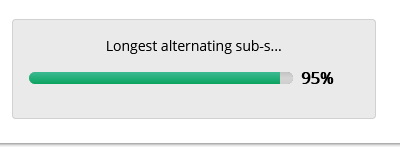## Problem Statement :

Consider a non-empty string instr containing

only lower case alphabets. Print the output

based on the below logic:

• Identify and print the longest sub-sequence of at least 3 alphabets alternating between vowels and consonants possible from instr. The sub-sequence can start with a vowel or a consonant

A sub-sequence of a string str is the possible set of characters formed from str starting from left to right

• If more than one such longest sub-sequences are identified, print the one having the highest sum of ASCII values of its alphabets

• If no such sub-sequence of at least 3 alphabets is possible, print X (uppercase)

## Question :## Code Solution :

```				```
def isVowel(c):
vowels=['a','e','i','o','u']
if(c in vowels):
return True
return False

def Subsequence(str):
ans=''
flag=(isVowel(str))
maxi=ord(str)

for i in range(1,len(str)):
if (isVowel(str[i]) == flag):
maxi=max(maxi,ord(str[i]))

else:
ans=ans+chr(maxi)
maxi=ord(str[i])

flag=not(flag)

ans=ans+chr(maxi)
return ans

#Driver program

input_string =input()
print(Subsequence(input_string))
```
```